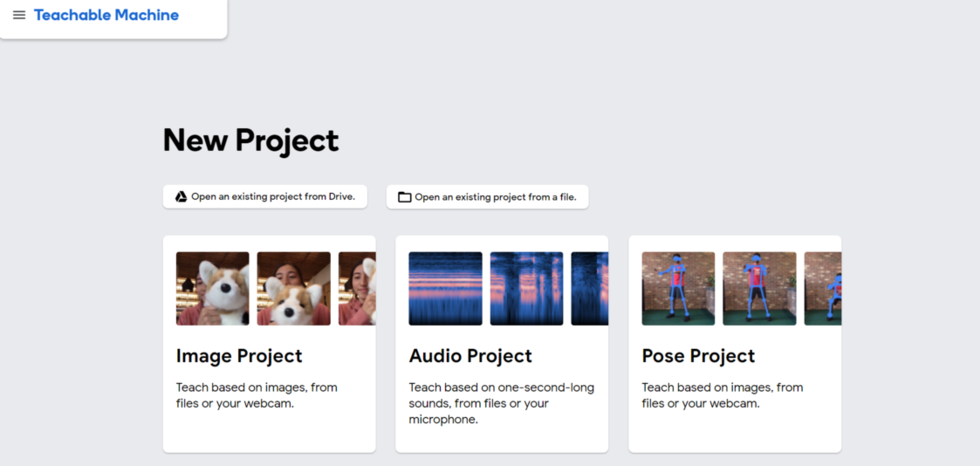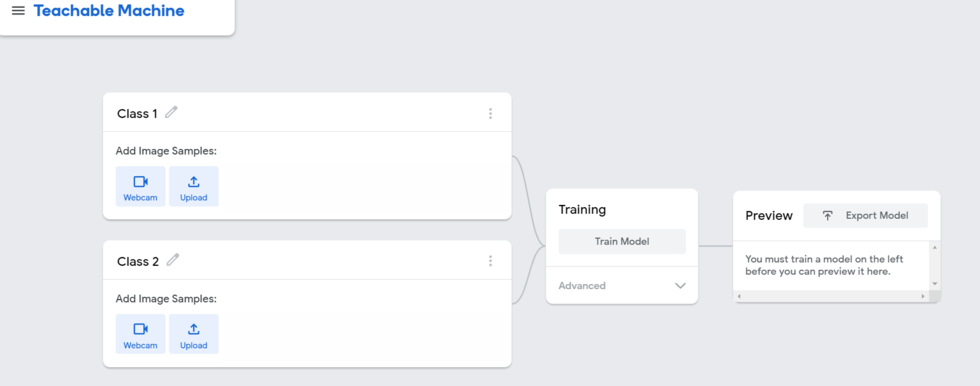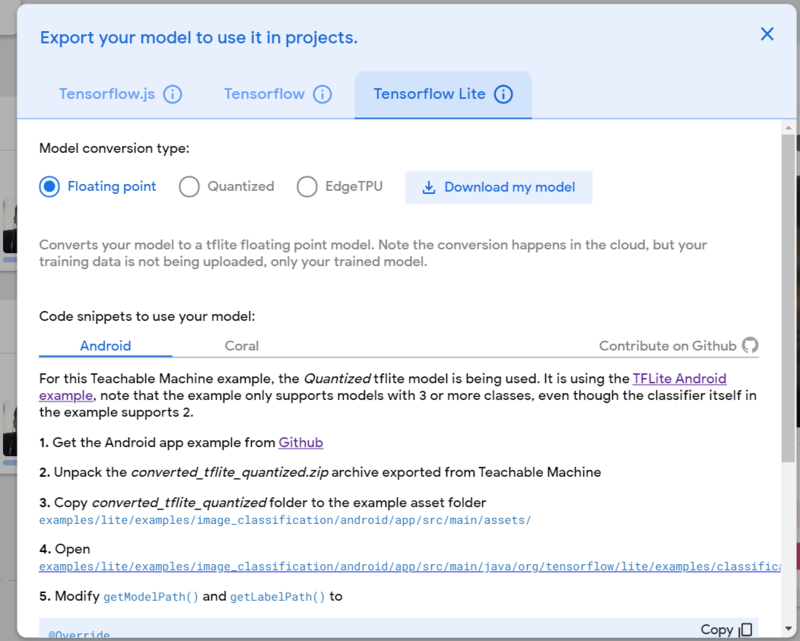Open in App
Not now

# Mask Detection App in Flutter

• Last Updated : 11 Jun, 2021

Nowadays in the situation of Covid, it is very important to wear a Mask and protect yourself and others. So this App helps to detect whether the person has worn the Mask or Not. In this Application, the Mask detection is done with the help of TensorFlow Lite.

• Add the dependency to pubspec.yaml file.
• Import the dependency to the main.dart file

Add the given dependency in pubspec.yaml file

## Dart

 `dependencies: ` `  `  `  ``cupertino_icons: ^1.0.2 ` `  ``image_picker: ^0.7.4 ` `  ``tflite: ` `  ``google_fonts:`

Step 2: Now navigate to main.dart() file and return Material App().

First, we have declared MyApp() in runApp in the main function. Then we have created StatelessWidget for MyApp in which we have returned MaterialApp(). In this MaterialApp() we have given the title of our App then declared the theme of our App as primaryColor as blue. Then we have given our first screen of or slider app in the home: HomePage()

## Dart

 `void` `main() { ` `  ``runApp(MyApp()); ` `} ` ` `  `class` `MyApp extends StatelessWidget { ` `   `  `  ``// This widget is the root of your application. ` `  ``@override ` `  ``Widget build(BuildContext context) { ` `     `  `    ``// Material App ` `    ``return` `MaterialApp( ` `      ``debugShowCheckedModeBanner: ``false``, ` `      ``title: ``'Flutter Demo'``, ` `      ``theme: ThemeData( ` `      ``brightness: Brightness.dark, ` `        ``primaryColor: Color(0xff01AEBC), ` `      ``), ` `       `  `      ``// Main Screen in our app ` `      ``home: HomePage(), ` `    ``); ` `  ``} ` `}`

Step 3: Now declare StatefulWidget() for HomePage() class.

In this StatefulWidget() we have declared various variable under state function. And after that we have declare methods for loadImageGallery() and loadImageCamera(). These methods are used for picking the images from the gallery and camera with the help of the image_picker library which we have imported.

## Dart

 `class` `HomePage extends StatefulWidget { ` `  ``@override ` `  ``_HomePageState createState() => _HomePageState(); ` `} ` ` `  `class` `_HomePageState extends State { ` `   `  `  ``// Variables Declared ` `  ``bool` `_loading = ``true``; ` `  ``File _image; ` `  ``final imagePicker = ImagePicker(); ` `  ``List _predictions = []; ` ` `  `  ``// Methods to pick the image  from Camera and Gallery ` `  ``Future loadImageGallery() async { ` `    ``final image = await imagePicker.getImage(source: ImageSource.gallery); ` `    ``detectImage(image); ` `  ``} ` ` `  `  ``Future loadImageCamera() async { ` `    ``final image = await imagePicker.getImage(source: ImageSource.camera); ` `    ``detectImage(image); ` `  ``}`

Step 4: Now Go On Google Teachable Machine.

Now Go On a Google Teachable Machine. Where you have o train the Model for Mask Detection. Now Click On Image Project. Here You have to create Two Classes One for Mask and the other for No Mask. Now in the Mask Class click on WebCam to record your face Mask from all Angles. In the same way you will record your face Without Mask in the No Mask Class from all Angles. Now click on Train Model.

After completing that you will click on Export Model.

Step 1:Step 2:Step 3:After that click on Tensorflow Lite and in that select Floating point and then click on Download my model. Now After Downloading the Model Extract the file. Now add the extracted files to the assets folder in pubspec.yaml file.

Now we have created loadModel() in this we have added extracted files of our downloaded file in this method. And we have initialized the loadModel() in initState(). After that, we have created detectImage() for detecting the image. After that, we have set the state to update the image.

And we have Ended the function using dispose().

## Dart

 `//Initialize the state  ` `@override ` `  ``void` `initState() { ` `    ``// TODO: implement initState ` `    ``super.initState(); ` `    ``loadModel(); ` `  ``} ` ` `  `  ``// Added extracted files in loadModel() function ` `  ``loadModel() async{ ` `    ``Tflite.close(); ` `    ``await Tflite.loadModel(model: ``'assets/model_unquant.tflite'``, ` `                           ``labels: ``'assets/labels.txt'``); ` `  ``} ` ` `  ` ``// Created detectImage() for detecting the image ` ` ``Future detectImage(image) async { ` `    ``var prediction = await Tflite.runModelOnImage( ` `        ``path: image.path, ` `        ``numResults: 2, ` `        ``threshold: 0.6, ` `        ``imageMean: 127.5, ` `        ``imageStd: 127.5, ` `    ``); ` `    ``setState(() { ` `      ``_loading = ``false``; ` `      ``if` `(image != null) { ` `        ``_image = File(image.path); ` `        ``_predictions = prediction; ` `      ``} ``else` `{ ` `        ``print(``'No image selected.'``); ` `      ``} ` `    ``}); ` `  ``} ` ` `  `  ``// Ended the function ` `  ``@override ` `  ``void` `dispose() { ` `    ``// TODO: implement dispose ` `    ``super.dispose(); ` `  ``} `

Step 6: After that, we have given the UI of our Application.

In this StateFulWidget() we have declared Scaffold(). In which we have declared Appbar() in this appbar we have given the title of our app in Text Widget. After the appbar in the body section, we have declared Column() in which the image and the buttons will appear one below another. When we click on Capture Image Button the camera of the device will open, and you have to capture the image that will detect the mask.

In the same way when you click on Pick Image from Gallery. Your File manager will open from where you can select Image which will be used for detecting the mask.

## Dart

 `@override ` `  ``Widget build(BuildContext context) { ` `    ``return` `Scaffold( ` `       `  `      ``// Appbar declared ` `      ``appBar: AppBar( ` `        ``title: Text(``'Mask Detection'``, ` `        ``style: GoogleFonts.robotoCondensed(), ` `        ``), ` `      ``), ` `      ``body: SingleChildScrollView( ` `         `  `        ``// Column containing images and buttons ` `        ``child: Column( ` `          ``crossAxisAlignment: CrossAxisAlignment.center, ` `          ``children: [ ` `             `  `            ``// Image will display here ` `            ``_loading == ``false` `? Padding( ` `              ``padding: ``const` `EdgeInsets.all(10.0), ` `              ``child: Container( ` `                ``child: Column( ` `                  ``children: [ ` `                    ``Container( ` `                      ``height: 280, ` `                      ``width: 220, ` `                      ``decoration: BoxDecoration( ` `                        ``borderRadius: BorderRadius.circular(25), ` `                      ``), ` `                      ``child: ClipRRect( ` `                          ``borderRadius: BorderRadius.circular(25), ` `                          ``child: Image.file(_image)), ` `                    ``), ` `                    ``SizedBox(height: 10), ` ` `  `                    ``Text(_predictions[``'label'``].toString().substring(2), ` `                      ``style: GoogleFonts.robotoCondensed( ` `                        ``fontSize: 20, ` `                      ``), ` `                    ``), ` `                    ``SizedBox(height: 10), ` `                    ``// Text('Safer: '+"\${(_predictions ` `                    ``// ['confidence']*100).toString()}%"), ` `                  ``], ` `                ``), ` `              ``), ` `            ``):Padding( ` `              ``padding: ``const` `EdgeInsets.all(10.0), ` `              ``child: Container( ` `                ``height: 300, ` `                ``width: 250, ` `                ``decoration: BoxDecoration( ` `                  ``borderRadius: BorderRadius.circular(15), ` `                  ``color: Colors.grey, ` `                ``), ` `                ``child: Center(child: Text(``'Image Appears here'``)), ` `              ``), ` `            ``), ` `            ``SizedBox(height: 10), ` `             `  `            ``// Capture Image from Camera ` `            ``Padding( ` `              ``padding: ``const` `EdgeInsets.all(10.0), ` `              ``child: Container( ` `                ``height: 50, ` `                ``width: ``double``.infinity, ` `                ``child: RaisedButton( ` `                    ``onPressed: (){ ` `                       `  `                     ``// Methods we created above ` `                      ``loadImageCamera(); ` `                    ``}, ` `                ``child: Text(``'Capture Image'``), ` `                  ``color: Color(0xff01AEBC), ` `                  ``shape: RoundedRectangleBorder( ` `                    ``borderRadius: BorderRadius.circular(15), ` `                  ``), ` `                ``), ` `              ``), ` `            ``), ` `            ``SizedBox(height: 10), ` `             `  `            ``// Capture Image from Gallery ` `            ``Padding( ` `              ``padding: ``const` `EdgeInsets.all(10.0), ` `              ``child: Container( ` `                ``height: 50, ` `                ``width: ``double``.infinity, ` `                ``child: RaisedButton( ` `                  ``onPressed: (){ ` `                     `  `                    ``// Methods we created above ` `                    ``loadImageGallery(); ` `                  ``}, ` `                  ``child: Text(``'Pick Image from Gallery'``), ` `                  ``color: Color(0xff01AEBC), ` `                  ``shape: RoundedRectangleBorder( ` `                    ``borderRadius: BorderRadius.circular(15), ` `                  ``), ` `                ``), ` `              ``), ` `            ``), ` `          ``], ` `        ``), ` `      ``), ` `    ``);`

Full Code for Mask Detection App:

## Dart

 `import ``'dart:io'``; ` `import ``'package:flutter/material.dart'``; ` `import ``'package:flutter/services.dart'``; ` `import ``'package:google_fonts/google_fonts.dart'``; ` `import ``'package:image_picker/image_picker.dart'``; ` `import ``'package:tflite/tflite.dart'``; ` ` `  `class` `HomePage extends StatefulWidget { ` `  ``@override ` `  ``_HomePageState createState() => _HomePageState(); ` `} ` ` `  `class` `_HomePageState extends State { ` `  ``bool` `_loading = ``true``; ` `  ``File _image; ` `  ``final imagePicker = ImagePicker(); ` `  ``List _predictions = []; ` `   `  `  ``// For picking image from Gallery ` `  ``Future loadImageGallery() async { ` `    ``final image = await imagePicker.getImage(source: ImageSource.gallery); ` `    ``detectImage(image); ` `  ``} ` `   `  `  ``// For picking image from Camera ` `  ``Future loadImageCamera() async { ` `    ``final image = await imagePicker.getImage(source: ImageSource.camera); ` `    ``detectImage(image); ` `  ``} ` ` `  `  ``@override ` `  ``void` `initState() { ` `    ``// TODO: implement initState ` `    ``super.initState(); ` `    ``loadModel(); ` `  ``} ` `   `  `  ``// LoadModel() for connection app with TensorFlow Lite ` `  ``loadModel() async{ ` `    ``Tflite.close(); ` `    ``await Tflite.loadModel(model: ``'assets/model_unquant.tflite'``, ` `                           ``labels: ``'assets/labels.txt'``); ` `  ``} ` `   `  ` ``// Method for Image detection ` ` ``Future detectImage(image) async { ` `    ``var prediction = await Tflite.runModelOnImage( ` `        ``path: image.path, ` `        ``numResults: 2, ` `        ``threshold: 0.6, ` `        ``imageMean: 127.5, ` `        ``imageStd: 127.5, ` `    ``); ` `    ``setState(() { ` `      ``_loading = ``false``; ` `      ``if` `(image != null) { ` `        ``_image = File(image.path); ` `        ``_predictions = prediction; ` `      ``} ``else` `{ ` `        ``print(``'No image selected.'``); ` `      ``} ` `    ``}); ` `    ``// setState(() { ` `    ``//   _loading = false; ` `    ``//   _predictions = prediction; ` `    ``// }); ` `  ``} ` `  ``@override ` `  ``void` `dispose() { ` `    ``// TODO: implement dispose ` `    ``super.dispose(); ` `  ``} ` ` `  ` `  `  ``@override ` `  ``Widget build(BuildContext context) { ` `    ``return` `Scaffold( ` `      ``// Appbar displayed here ` `      ``appBar: AppBar( ` `        ``title: Text(``'Mask Detection'``, ` `        ``style: GoogleFonts.robotoCondensed(), ` `        ``), ` `      ``), ` `      ``body: SingleChildScrollView( ` `        ``child: Column( ` `          ``crossAxisAlignment: CrossAxisAlignment.center, ` `          ``children: [ ` `             `  `             ``// Image will display here ` `            ``_loading == ``false` `? Padding( ` `              ``padding: ``const` `EdgeInsets.all(10.0), ` `              ``child: Container( ` `                ``child: Column( ` `                  ``children: [ ` `                    ``Container( ` `                      ``height: 280, ` `                      ``width: 220, ` `                      ``decoration: BoxDecoration( ` `                        ``borderRadius: BorderRadius.circular(25), ` `                      ``), ` `                      ``child: ClipRRect( ` `                          ``borderRadius: BorderRadius.circular(25), ` `                          ``child: Image.file(_image)), ` `                    ``), ` `                    ``SizedBox(height: 10), ` ` `  `                    ``Text(_predictions[``'label'``].toString().substring(2), ` `                      ``style: GoogleFonts.robotoCondensed( ` `                        ``fontSize: 20, ` `                      ``), ` `                    ``), ` `                    ``SizedBox(height: 10), ` `                    ``// Text('Safer: '+"\${(_predictions['confidence']*100).toString()}%"), ` `                  ``], ` `                ``), ` `              ``), ` `            ``):Padding( ` `              ``padding: ``const` `EdgeInsets.all(10.0), ` `              ``child: Container( ` `                ``height: 300, ` `                ``width: 250, ` `                ``decoration: BoxDecoration( ` `                  ``borderRadius: BorderRadius.circular(15), ` `                  ``color: Colors.grey, ` `                ``), ` `                ``child: Center(child: Text(``'Image Appears here'``)), ` `              ``), ` `            ``), ` `            ``SizedBox(height: 10), ` `            ``// Button for taking Image from Camera ` `            ``Padding( ` `              ``padding: ``const` `EdgeInsets.all(10.0), ` `              ``child: Container( ` `                ``height: 50, ` `                ``width: ``double``.infinity, ` `                ``child: RaisedButton( ` `                    ``onPressed: (){ ` `                      ``loadImageCamera(); ` `                    ``}, ` `                ``child: Text(``'Capture Image'``), ` `                  ``color: Color(0xff01AEBC), ` `                  ``shape: RoundedRectangleBorder( ` `                    ``borderRadius: BorderRadius.circular(15), ` `                  ``), ` `                ``), ` `              ``), ` `            ``), ` `            ``SizedBox(height: 10), ` `            ``// Button for taking Image from Gallery ` `            ``Padding( ` `              ``padding: ``const` `EdgeInsets.all(10.0), ` `              ``child: Container( ` `                ``height: 50, ` `                ``width: ``double``.infinity, ` `                ``child: RaisedButton( ` `                  ``onPressed: (){ ` `                    ``loadImageGallery(); ` `                  ``}, ` `                  ``child: Text(``'Pick Image from Gallery'``), ` `                  ``color: Color(0xff01AEBC), ` `                  ``shape: RoundedRectangleBorder( ` `                    ``borderRadius: BorderRadius.circular(15), ` `                  ``), ` `                ``), ` `              ``), ` `            ``), ` `          ``], ` `        ``), ` `      ``), ` `    ``); ` `  ``} ` `} `

Output:

My Personal Notes arrow_drop_up
Related Articles